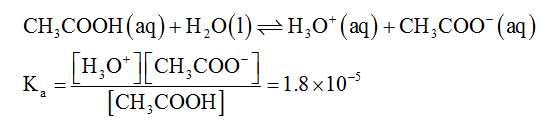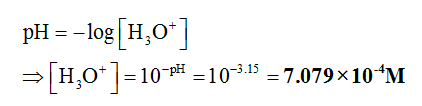# Specifically, the question is "If acetic acid is the only acid that vinegar contains (Ka = 1.8 x 10-5) calculate the concentration of acetic acid in the vinegar." "Given pH = 3.15"

Question
20 views

Specifically, the question is "If acetic acid is the only acid that vinegar contains (Ka = 1.8 x 10-5) calculate the concentration of acetic acid in the vinegar." "Given pH = 3.15"

check_circle

Step 1

The dominant equilibrium reaction is given below.The concentration of hydronium ion can be calculated from pH....

### Want to see the full answer?

See Solution

#### Want to see this answer and more?

Solutions are written by subject experts who are available 24/7. Questions are typically answered within 1 hour.*

See Solution
*Response times may vary by subject and question.
Tagged in